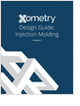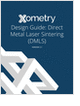MaterialsDesign CenterProcessesUnit ConversionFormulasMathematicsCalculatorsDiscussion ForumTrade PublicationsDirectory Service
Injection Molding Design Guide

Guide for high quality and cost-effective plastic injection molding.

3D Scanners

A white paper to assist in the evaluation of 3D scanning hardware solutions.

Metal 3D Printing Design Guide

Direct Metal Laser Sintering (DMLS) 3D printing for parts with reduced cost and little waste.

CNC Machining Design Guide

more free magazinesGlossary » Units » Thermal Conductivity » Watt Per Meter Per Celsius DegreeWatt Per Meter Per Celsius Degree (W/m-°C) is a unit in the category of Thermal conductivity. It is also known as watts per meter per Celsius degree, watt per metre per Celsius degree, watts per metre per Celsius degree. Watt Per Meter Per Celsius Degree (W/m-°C) has a dimension of MLT-3Q-1 where M is mass, L is length, T is time, and Q is temperatur. It essentially the same as the corresponding standard SI unit W/m-K.
Note that the seven base dimensions are M (Mass), L (Length), T (Time), Q (Temperature), N (Aamount of Substance), I (Electric Current), and J (Luminous Intensity).

Other units in the category of Thermal conductivity include British Thermal Unit (IT) Per Foot Per Hour Per Fahrenheit Degree (Btu (IT)/ft-h-°F), British Thermal Unit (IT)-Inch Per Square Foot Per Hour Per Fahrenheit Degree (Btu (IT)-in/h-ft2-°F), British Thermal Unit (IT)-Inch Per Square Foot Per Second Per Fahrenheit Degree (Btu (IT)-in/s-ft2-°F), British Thermal Unit (therm.) Per Foot Per Hour Per Fahrenheit Degree (Btu (therm.)/ft-h-°F), British Thermal Unit (therm.)-Inch Per Square Foot Per Hour Per Fahrenheit Degree (Btu (therm.)-in/h-ft2-°F), British Thermal Unit (therm.)-Inch Per Square Foot Per Second Per Fahrenheit Degree (Btu (therm.)-in/s-ft2-°F), Calorie (IT) Per Centimeter Per Second Per Degree Celsius (cal (IT)/cm-s-°C), Calorie (therm.) Per Centimeter Per Second Per Degree Celsius (cal (therm.)/cm-s-°C), Watt Per Centimeter Per Celsius Degree (W/cm-°C), and Watt Per Meter Per Kelvin (W/m-K).N/ARelated PagesGlossaryInjection Molding Design Guide

Guide for high quality and cost-effective plastic injection molding.3D Scanners

A white paper to assist in the evaluation of 3D scanning hardware solutions.Metal 3D Printing Design Guide

Direct Metal Laser Sintering (DMLS) 3D printing for parts with reduced cost and little waste.CNC Machining Design Guide